# Matching Supply with Demand

## Quiz 13 : Service Levels and Lead Times in Supply Chains: the Order Up-To Inventory ModelLooking for Management Homework Help?

## Quiz 13 :Service Levels and Lead Times in Supply Chains: the Order Up-To Inventory Model

Question Type(Furniture Store) You are the store manager at a large furniture store. One of your products is a study desk. Weekly demand for the desk is normally distributed with mean 40 and standard deviation 20. The lead time from the assembly plant to your store is two weeks and you order inventory replenishments weekly. You use the order-up-to model to control inventory. a. Suppose your order-up-to level is S = 220. You are about to place an order and note that your inventory level is 100 and you have 85 desks on order. How many desks will you order b. Suppose your order-up-to level is S = 220. You are about to place an order and note that your inventory level is 160 and you have 65 desks on order. How many desks will you order c. What is the optimal order-up-to level if you want to target a 98 percent in-stock probability d. Suppose your order-up-to level is S = 120. What is your expected on-hand inventory e. Suppose your order-up-to level is S = 120. Your internal cost of capital is 15 percent and each desk costs $200. What is your total cost of capital for the year for inventory in the store Free Essay Answer: Answer: The order-up-to-level inventory model is a fixed time period model where the review is carried out after a fixed interval of time. The order quantity depends on the existing inventory, scheduled receipts, and the protection level i.e. the order-up-to-level. In other words, the existing inventory level and scheduled order receipts are subtracted from the order-up-to-level to find the order quantity. a. Note that the order-up-to level, S = 220.So, the number of desks to be ordered =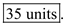b. Note that the order-up-to level, S = 220.So, the number of desks to be ordered =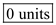as the inventory level plus the scheduled receipt is more than S. c. The average weekly demand,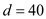The standard deviation of weekly demand,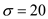Replenishment lead time,Review period,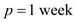Service level is 98%. So, the corresponding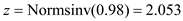Since fractional value for order-up-to level is infeasible, the optimal order-up-to level is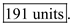d.The order-up-to level, S = 120. So, the value of z -statistic can be computed as: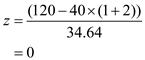The corresponding loss function value,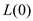from the table is 0.3989. Therefore,Since fractional value for on-hand inventory is infeasible, the on-hand inventory is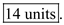e.The order-up-to level, S = 120. So, the value of z -statistic can be computed as:The corresponding loss function value,from the table is 0.3989. Therefore,So, the annual cost of capital blocked for inventory is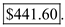Tags(Campus Bookstore) A campus bookstore sells the Palm m505 handheld for$399. The wholesale price is $250 per unit. The store estimates that weekly demand averages 0.5 unit and has a Poisson distribution. The bookstore's annual inventory holding cost is 20 percent of the cost of inventory. Assume orders are made weekly and the lead time to receive an order from the distributor is four weeks. a. What base stock level minimizes inventory while achieving a 99 percent in-stock probability b. Suppose the base stock level is S = 4. What is the average pipeline inventory c. Suppose the base stock level is S = 5. What is the average inventory held at the end of the week in the store d. Suppose the base stock level is S = 6. What is the probability a stockout occurs during a week (i.e., some customer is back-ordered) e. Suppose the base stock level is S = 6. What is the probability the store is out of stock (i.e., has no inventory) at the end of a week f. Suppose the base stock level is S = 6. What is the probability the store has one or more units of inventory at the end of a week The bookstore is concerned that it is incurring excessive ordering costs by ordering weekly. For parts g and h, suppose the bookstore now submits orders every two weeks. The demand forecast remains the same and the lead time is still four weeks. g. What base stock level yields at least a 99 percent in-stock probability while minimizing inventory h. What is the average pipeline stock Free Essay Answer: Answer: In a single-period inventory model, the order size decision is made by a marginal analysis. In this analysis, the optimal order quantity occurs at the point where the marginal benefit of stocking one additional unit is just less than its expected marginal cost. a. The mean demand over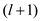periods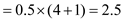units In-stock probability = 99% From the Poisson distribution table, for mean equal to 2.5,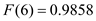and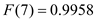. Since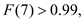the optimal order quantity is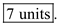b. The pipeline inventory is computed by multiplying the lead time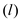by the expected demand in one period.So, the average pipeline inventory is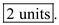c. Base stock level, S = 5 From the Poisson table, for mean equal to 2.5,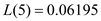So, the expected inventory held at the end of the week is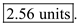d. Base stock level, S = 6 From the Poisson distribution table, for mean equal to 2.5,So, the in-stock probability is 0.9858 or 98.58% Therefore, the probability of stock out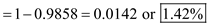e. There will be stockout at the end of week when the demand for theperiods is less than S = 6. From the Poisson distribution table, for mean equal to 2.5,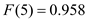So, the probability of stockoutSo, the probability of stockout at the end of the period is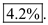f. The probability that there will be one or more units at the end of weekSo, the probability that there will be one or more units at the end of week is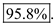g. Average lead time,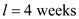Review and ordering period,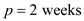The mean demand over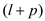periods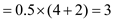units In-stock probability = 99% From the Poisson distribution table, for mean equal to 3,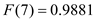and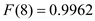Since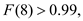the optimal order quantity is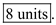h.So, the average pipeline inventory isTags(Quick Print) Quick Print Inc. uses plain and three-hole-punched paper for copying needs. Demand for each paper type is highly variable. Weekly demand for the plain paper is estimated to be normally distributed with mean 100 and standard deviation 65 (measured in boxes). Each week, a replenishment order is placed to the paper factory and the order arrives five weeks later. All copying orders that cannot be satisfied immediately due to the lack of paper are back-ordered. The inventory holding cost is about$1 per box per year. a. Suppose that Quick Print decides to establish an order-up-to level of 700 for plain paper. At the start of this week, there are 523 boxes in inventory and 180 boxes on order. How much will Quick Print order this week b. What is Quick Print's optimal order-up-to level for plain paper if Quick Print operates with a 99 percent in-stock probability
Free
Essay

The order-up-to-level inventory model is a fixed time period model where the review is carried out after a fixed interval of time. The order quantity depends on the existing inventory, scheduled receipts, and the protection level i.e. the order-up-to-level. In other words, the existing inventory level and scheduled order receipts are subtracted from the order-up-to-level to find the order quantity.
Consider the data given below:
The average weekly demand,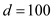The standard deviation of weekly demand,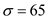Replenishment lead time,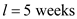Review period,a.
Note that the order-up-to level, S = 700.So, the number of units to be ordered =as the inventory level plus the scheduled receipt is more than S.
b.
Service level is 99%. So, the corresponding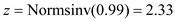Since fractional value for order-up-to level is infeasible, the optimal order-up-to level is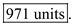Tags(Main Line Auto Distributor) Main Line Auto Distributor is an auto parts supplier to local garage shops. None of its customers have the space or capital to store all of the possible parts they might need so they order parts from Main Line several times a day. To provide fast service, Main Line uses three pickup trucks to make its own deliveries. Each Friday evening, Main Line orders additional inventory from its supplier. The supplier delivers early Monday morning. Delivery costs are significant, so Main Line only orders on Fridays. Consider part A153QR, or part A for short. Part A costs Main Line $175 and Main Line sells it to garages for$200. If a garage orders part A and Main Line is out of stock, then the garage finds the part from some other distributor. Main Line has its own capital and space constraints and estimates that each unit of part A costs $0.50 to hold in inventory per week. (Assume you incur the$0.50 cost for units left in inventory at the end of the week, not $0.50 for your average inventory during the week or$0.50 for your inventory at the start of the week.) Average weekly demand for this part follows a Poisson distribution with mean 1.5 units. Suppose it is Friday evening and Main Line currently doesn't have any part A's in stock. The distribution and loss functions for a Poisson distribution with mean 1.5 can be found in Appendix B. a. How many part A's should Main Line order from the supplier b. Suppose Main Line orders three units. What is the probability Main Line is able to satisfy all demand during the week c. Suppose Main Line orders four units. What is the probability Main Line is not able to satisfy all demand during the week d. If Main Line seeks to hit a target in-stock probability of 99.5 percent, then how many units should Main Line order e. Suppose Main Line orders five units. What is Main Line's expected holding cost for the upcoming week
Essay
Tags(Hotspices.com) You are the owner of Hotspices.com, an online retailer of hip, exotic, and hard-to-find spices. Consider your inventory of saffron, a spice (generally) worth more by weight than gold. You order saffron from an overseas supplier with a shipping lead time of four weeks and you order weekly. Average quarterly demand is normally distributed with a mean of 415 ounces and a standard deviation of 154 ounces. The holding cost per ounce per week is $0.75. You estimate that your back-order penalty cost is$50 per ounce. Assume there are 4.33 weeks per month. a. If you wish to minimize inventory holding costs while maintaining a 99.25 percent instock probability, then what should your order-up-to level be b. If you wish to minimize holding and back-order penalty costs, then what should your order-up-to level be c. Now consider your inventory of pepperoncini (Italian hot red peppers). You can order this item daily and your local supplier delivers with a two-day lead time. While not your most popular item, you do have enough demand to sell the five-kilogram bag. Average demand per day has a Poisson distribution with mean 1.0. The holding cost per bag per day is $0.05 and the back-order penalty cost is about$5 per bag. What is your optimal order-up-to level
Essay
Tags(Blood Bank) Dr. Jack is in charge of the Springfield Hospital's Blood Center. Blood is collected in the regional Blood Center 200 miles away and delivered to Springfield by airplane. Dr. Jack reviews blood reserves and places orders every Monday morning for delivery the following Monday morning. If demand begins to exceed supply, surgeons postpone nonurgent procedures, in which case blood is back-ordered. Demand for blood on every given week is normal with mean 100 pints and standard deviation 34 pints. Demand is independent across weeks. a. On Monday morning, Dr. Jack reviews his reserves and observes 200 pints in on-hand inventory, no back orders, and 73 pints in pipeline inventory. Suppose his order-up-to level is 285. How many pints will he order Choose the closest answer. b. Dr. Jack targets a 99 percent in-stock probability. What order-up-to level should he choose Choose the closest answer. c. Dr. Jack is planning to implement a computer system that will allow daily ordering (seven days per week) and the lead time to receive orders will be one day. What will be the average order quantity
Essay
Tags(Schmears Shirts) Schmears Inc. is a catalog retailer of men's shirts. Daily demand for a particular SKU (style and size) is Poisson with mean 1.5. It takes three days for a replenishment order to arrive from Schmears' supplier and orders are placed daily. Schmears uses the order-up-to model to manage its inventory of this shirt. a. Suppose Schmears uses an order-up-to level of 9. What is the average number of shirts on order b. Now suppose Schmears uses an order-up-to level of 8. What is the probability during any given day that Schmears does not have sufficient inventory to meet the demand from all customers c. Suppose Schmears wants to ensure that 90 percent of customer demand is satisfied immediately from stock. What order-up-to level should they use d. Schmears is considering a switch from a "service-based" stocking policy to a "cost-minimization" stocking policy. They estimate their holding cost per shirt per day is $0.01. Forty-five percent of customers order more than one item at a time, so they estimate their stockout cost on this shirt is$6 per shirt. What order-up-to level minimizes the sum of their holding and back-order costs
Essay
Tags(ACold) ACold Inc. is a frozen food distributor with 10 warehouses across the country. Iven Tory, one of the warehouse managers, wants to make sure that the inventory policies used by the warehouse are minimizing inventory while still maintaining quick delivery to ACold's customers. Since the warehouse carries hundreds of different products, Iven decided to study one. He picked Caruso's Frozen Pizza. Demand for CFPs averages 400 per day with a standard deviation of 200. Weekly demand (five days) averages 2,000 units with a standard deviation of 555. Since ACold orders at least one truck from General Foods each day (General Foods owns Caruso's Pizza), ACold can essentially order any quantity of CFP it wants each day. In fact, ACold's computer system is designed to implement a base stock policy for each product. Iven notes that any order for CFPs arrives four days after the order. Further, it costs ACold $0.01 per day to keep a CFP in inventory, while a back order is estimated to cost ACold$0.45. a. What base stock level should Iven choose for CFPs if his goal is to minimize holding and back-order costs b. Suppose the base stock level 2,800 is chosen. What is the average amount of inventory on order c. Suppose the base stock level 2,800 is chosen. What is the annual holding cost (Assume 260 days per year.) d. What base stock level minimizes inventory while maintaining a 97 percent in-stock probability
Essay(Cyber Chemicals) Cyber Chemicals uses liquid nitrogen on a regular basis. Average daily demand is 178 gallons with a standard deviation of 45. Due to a substantial ordering cost, which is estimated to be $58 per order (no matter the quantity in the order), Cyber currently orders from its supplier on a weekly basis. Cyber also incurs holding costs on its inventory. Cyber recognizes that its inventory is lowest at the end of the week but prefers a more realistic estimate of its average inventory. In particular, Cyber estimates its average inventory to be its average end-of-week inventory plus half of its average order quantity. The holding cost Cyber incurs on that average inventory is$0.08 per gallon per week. Cyber's supplier delivers in less than a day. Assume 52 weeks per year, five days per week. a. Cyber wishes to maintain a 99.9 percent in-stock probability. If it does so, what is Cyber's annual inventory holding cost b. What is Cyber's annual ordering cost c. Should Cyber consider ordering every two weeks